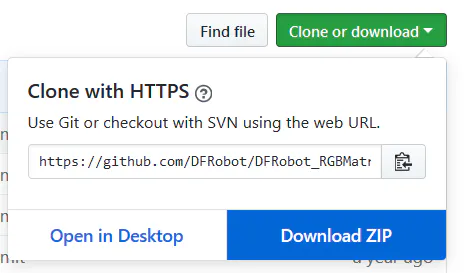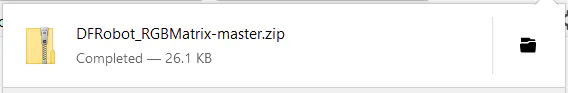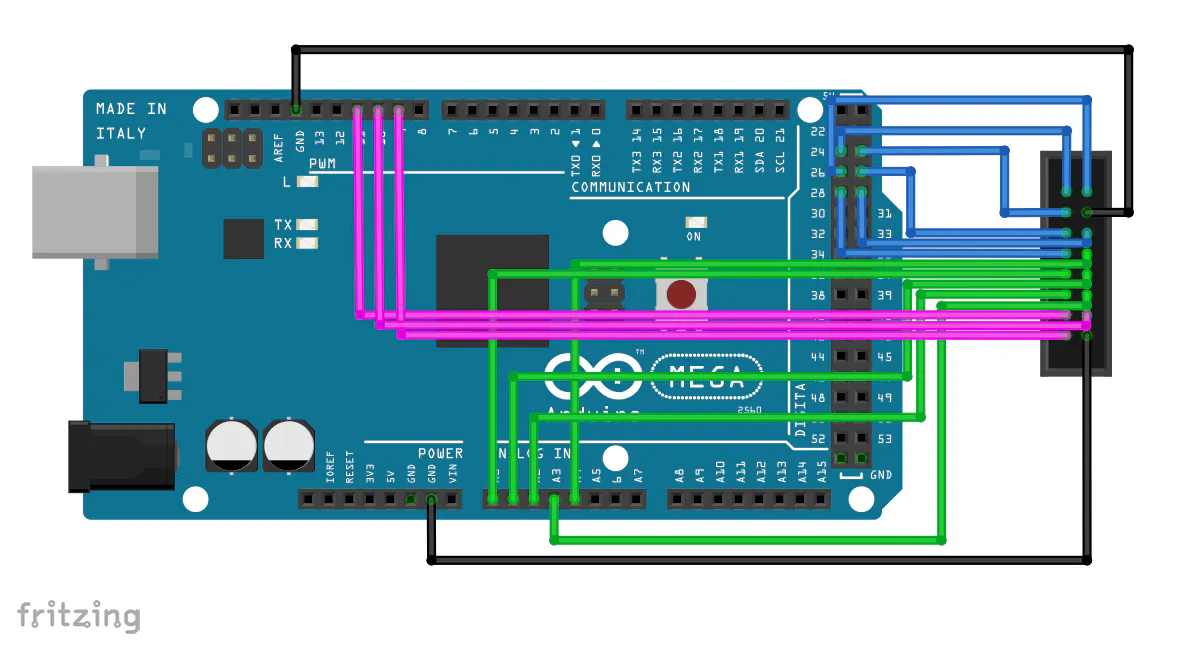PROJECTS Arduino

## Using the DFRobot RGB LED Matrix

DFRobot Oct 08 2018 1021

## Story

#### First, you'll need to set up the Mega 2560 with the proper library. Go to: https://github.com/DFRobot/DFRobot_RGBMatrix and download the library as a ZIP.### Schematics### Code

```/* Code by Maddy McGee
* Updated 7/2/18
*
* Results: White text that says "LED MATRIX" stays on the screen for 2000ms.
* Then the text changes color through the rainbow and moves from the bottom of the screen to the top of the screen.
*/
#include <DFRobot_RGBMatrix.h> // Hardware-specific library

//variables for all DFRobot RGB Matrix programs
#define OE    9
#define LAT   10
#define CLK   11
#define A     A0
#define B     A1
#define C     A2
#define D     A3
#define E     A4
#define WIDTH 64
#define HEIGHT  64

DFRobot_RGBMatrix matrix(A, B, C, D, E, CLK, LAT, OE, false, WIDTH, HEIGHT);

//variables for this specific program
boolean isDown;//determines if the text is moving down
int y;//y position of the text
int c;//counter for changing text color
uint16_t text;//the text color
const uint16_t BACK = matrix.Color333(0, 0, 0);//the background color is black

void setup() {
matrix.begin();
text = matrix.Color333(7, 7, 7); //set text color to white
isDown = true;//text is moving down at start
y = 28;//text starts in the middle

// fill with the background color
matrix.fillScreen(BACK);

// format text and display before starting motion
matrix.setTextSize(1);// size 1 is 8 'pixels' or LEDs tall
matrix.setTextColor(text);
matrix.setCursor(2, y);
matrix.setTextColor(text);
matrix.println("LED MATRIX");

delay(2000);
}

void loop() {
//clear previous text
matrix.setCursor(2, y);
matrix.setTextColor(BACK);
matrix.println("LED MATRIX");

//move next text
if (isDown){
if(y<56){
y++;
}else{
isDown = false;
}
}else{
if(y>0){
y--;
}else{
isDown = true;
}
}

//change color for next text
if(c<43){
c++;
} else{
c = 0;
}
changeTextColor(c);

//print new text
matrix.setCursor(2, y);
matrix.setTextColor(text);
matrix.println("LED MATRIX");

delay(150);
}

// Changes variable text to a color based on input integer from 0 to 43
// If the input is to great color is white, if the input is too low color is black
// As the input increments the colors change red, yellow, green, cyan, blue, magenta, back to almost red
void changeTextColor(int pos) {
if(pos<0){
//negative input, black
text = matrix.Color333(0, 0, 0);
} else if(pos < 7) {
//red to yellow
text = matrix.Color333(7, pos, 0);
} else if(pos < 15) {
//yellow to green
text = matrix.Color333(14-pos, 7, 0);
} else if(pos < 22) {
//green to cyan
text = matrix.Color333(0, 7, pos-14);
} else if(pos < 30){
//cyan to blue
text = matrix.Color333(0, 29-pos, 7);
} else if(pos < 37){
//blue to magenta
text = matrix.Color333(pos-29, 0, 7);
} else if(pos < 44){
//magenta to red
text = matrix.Color333(7, 0, 44-pos);
} else {
//input out of bounds, white
text = matrix.Color333(7, 7, 7);
}
}```